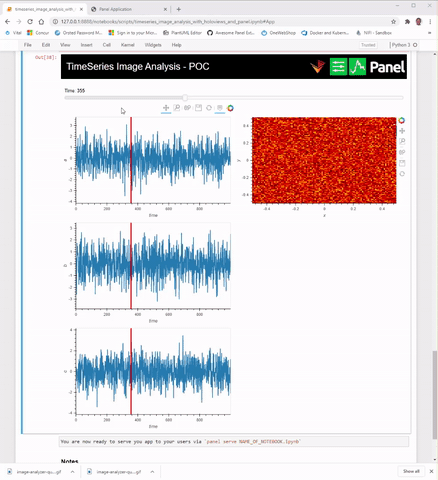# DynamicMaps with custom panel layouts

Just wondering if there’s a guide that shows how to use DynamicMaps with custom Panel layouts?

In particular, we have three components we need to scrub through, one is a video, the other two are timeseries plots for which we have a dynamic map VLine that shows us at which time step we’re at. We want to put the video on the right, the timeseries plots on the left, and the scrubber at the bottom, while taking advantage of the syntactic ease and composability of using DynamicMaps.

I put up a reprex here: https://gist.github.com/ericmjl/3d05c15339bd0cd26e07165b43035e7a.

Hi @ericmjl

I’ve attached an example. I chose to refactor a bit because my Panel skills are stronger than HoloViews skills.

I don’t know if it works exactly as you would like. But let me know if there are more questions.2 Likes

I believe the speed/ performance of the above could be improved if the 3 plots could be a HoloMap. I simply could not get access to the slider. If I could I could have watched the slider value for changes and update the image only when the slider changes. That should have been faster I believe.

I’d agree with @Marc that constructing the widget with Panel is the simpler approach here, however with sufficient indexing and some linking you can achieve something similar by using HoloViews:

``````left = pn.panel(img.opts(axiswise=True))
right = pn.panel((plot1+plot2+plot3).cols(1))

# We index the widgets from both plots and link them

pn.Column(
right,
pn.Row(left, right)
)
``````

Basically to achieve this we use `pn.panel` to convert our HoloViews objects to Panel objects and then index them so we get access to the widgets and plot components and then link and lay them out manually.

That said there’s also a much more efficient way to construct @Marc’s example, in that HoloViews DynamicMaps accept callbacks annotated with `pn.depends` and then efficiently update the plots. That would look like this, building on his existing code:

``````frame_slider = pn.widgets.IntSlider(name="Time", value=0, start=0, end=999)

@pn.depends(frame=frame_slider.param.value)
def image_cb(frame):
return get_image(frame).opts(responsive=True)

@pn.depends(frame=frame_slider.param.value)
def plots_cb(frame):
return get_plots(frame)

img_dmap = hv.DynamicMap(image_cb)
plots_dmap = hv.DynamicMap(plots_cb)

app = pn.Column(
app_bar,
pn.Spacer(height=10),
frame_slider,
pn.Row(
plots_dmap,
pn.Column(
pn.Spacer(height=20),
img_dmap,
),
),
)
app.servable()
``````

Thanks so much @philippjfr. I think these concepts are really powerfull.

But is there a way (holomap) in which I can have the three time series plots and all their data in the browser and the only callback is to the image?

My hope is that it could speed things up a bit. My hypothesis is that too much data is transferred and redrawn currently.

But is there a way (holomap) in which I can have the three time series plots and all their data in the browser and the only callback is to the image?

Oh right yes, missed that part. That is of course possible:

``````frame_slider = pn.widgets.IntSlider(name="Time", value=0, start=0, end=999)

plot_a = ts_data.hvplot(y="a", responsive=True, height=HEIGHT)
plot_b = ts_data.hvplot(y="b", responsive=True, height=HEIGHT)
plot_c = ts_data.hvplot(y="c", responsive=True, height=HEIGHT)

@pn.depends(frame=frame_slider)
def get_vline(frame):
return hv.VLine(frame).opts(color="red")

vline_dmap = hv.DynamicMap(get_vline)

((plot_a + plot_b + plot_c) * vline_dmap).cols(1)
``````

So the whole example should be:

``````frame_slider = pn.widgets.IntSlider(name="Time", value=0, start=0, end=999)

@pn.depends(frame=frame_slider.param.value)
def image_cb(frame):
return get_image(frame_slider.value).opts(responsive=True)

plot_a = ts_data.hvplot(y="a", responsive=True, height=HEIGHT)
plot_b = ts_data.hvplot(y="b", responsive=True, height=HEIGHT)
plot_c = ts_data.hvplot(y="c", responsive=True, height=HEIGHT)

@pn.depends(frame=frame_slider)
def get_vline(frame):
return hv.VLine(frame).opts(color="red")

vline_dmap = hv.DynamicMap(get_vline)
img_dmap = hv.DynamicMap(image_cb)

plots = ((plot_a + plot_b + plot_c) * vline_dmap).cols(1)

app = pn.Column(
app_bar,
pn.Spacer(height=10),
frame_slider,
pn.Row(
plots,
pn.Column(
pn.Spacer(height=20),
img_dmap,
),
),
)
app.servable()``````
1 Like

FYI @ericmjl and others. I’ve updated the notebook (attached below) based on @philippjfr suggestions.

It makes the app much more fast and responsive. It’s really, really nice now.

Please try it out and let me know if it works for you.ps. I somebody could point me to a real data set for which the tool could provide some actual insights it would be really, really nice. And we could add it to the Panel Gallery I believe to help new users.

pps. I’ve added the notebook as a PR to Panel here https://github.com/holoviz/panel/pull/1576